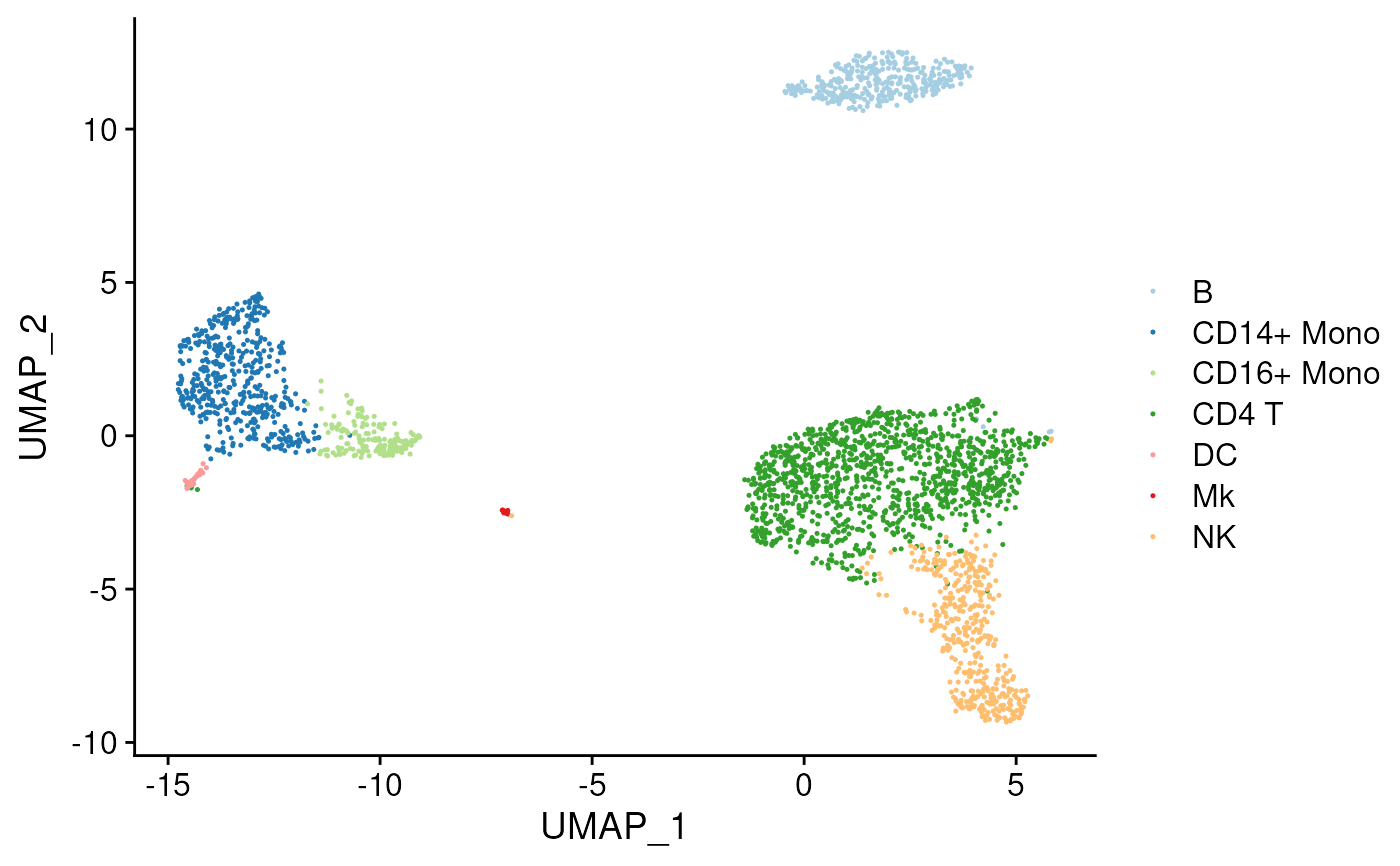Plot best calls for each cluster on a tSNE or umap

## Usage

plot_best_call(
cor_mat,
cluster_col = "cluster",
collapse_to_cluster = FALSE,
threshold = 0,
x = "UMAP_1",
y = "UMAP_2",
plot_r = FALSE,
per_cell = FALSE,
...
)

## Arguments

cor_mat

input similarity matrix

input metadata with tsne or umap coordinates and cluster ids

cluster_col

metadata column, can be cluster or cellid

collapse_to_cluster

if a column name is provided, takes the most frequent call of entire cluster to color in plot

threshold

minimum correlation coefficent cutoff for calling clusters

x

x variable

y

y variable

plot_r

whether to include second plot of cor eff for best call

per_cell

whether the cor_mat was generate per cell or per cluster

...

passed to plot_dims

## Value

ggplot object, cells projected by dr, colored by cell type classification

## Examples

res <- clustify(
input = pbmc_matrix_small,
ref_mat = cbmc_ref,
query_genes = pbmc_vargenes,
cluster_col = "classified"
)
#> using # of genes: 599
#> similarity computation completed, matrix of 9 x 13, preparing output

plot_best_call(
cor_mat = res,